# Fourier equation for given part of cos function between -pi and pi

#### Michael Zerafa

Joined Mar 22, 2007
4
I was following a post by SM in another section related to Networking but had no feedback. I am trying my luck in this section as the title is more related to Maths than electronics at the stage.

Question and a0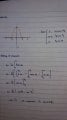an, bn, f(x) and plot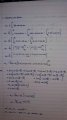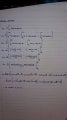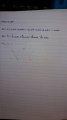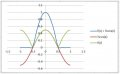Following the general equation as quoted from various reputable sources, such as John Bird,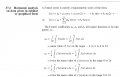although the cos(x)/2 term makes perfect sense, I cannot understand its origin. I have proved it graphically to be the missing term, but mathematically I cannot.

Please follow my reasoning as I am confident in my working but I am just as convinced I am missing something fundamental.

Thanks for your help so far
Mike

ps. the graph x axis is plotted as a function of pi, i.e. 1 is actually pi, 0.5 is pi/2

#### MrAl

Joined Jun 17, 2014
10,621
I was following a post by SM in another section related to Networking but had no feedback. I am trying my luck in this section as the title is more related to Maths than electronics at the stage.

Question and a0

View attachment 134443

an, bn, f(x) and plot
View attachment 134444 View attachment 134445 View attachment 134446 View attachment 134447

Following the general equation as quoted from various reputable sources, such as John Bird, View attachment 134448 although the cos(x)/2 term makes perfect sense, I cannot understand its origin. I have proved it graphically to be the missing term, but mathematically I cannot.

Please follow my reasoning as I am confident in my working but I am just as convinced I am missing something fundamental.

Thanks for your help so far
Mike

ps. the graph x axis is plotted as a function of pi, i.e. 1 is actually pi, 0.5 is pi/2
Hi Mike,

and the simple answer is that when we calculate the coefficient for a1 we have to use the concept of limits as we can not calculate it directly because we get zero in the denominator.

If you are also asking or instead asking about a0, then we can do the same thing using 'an' and then we might again have to resort to limits in order to calculate a0. So a0 can come from the usual expression for a0 or from the 'an' by either setting n=0 if that works, or taking the limit as n goes to zero. The calculation is similar to doing a1 and is shown in that Homework section where you had asked about a1 previously.

•Saviour Muscat